#DAY 4
0
AI & Data

## 前言

1. 為什麼模型要設成兩層完全連接層(Dense)?
2. 為什麼第一層完全連接層(Dense)要設為 128?
3. activation function、優化器(optimizer)、損失函數(loss)、效能衡量指標(metrics) 要如何選擇?
4. 為什麼使用小畫家，寫0~9實際測試，準確率好像不如測試資料那麼高? 我寫9，永遠辨識錯誤，唉 !
5. 使用像素辨識，好像不是很高明，應該用輪廓或線條比較好吧。
6. 如果要辨識多個數字，例如車牌，要怎麼作?
7. 如果要辨識其他事物，怎麼作?

## 為什麼模型要設成兩層完全連接層(Dense)?

``````# 建立模型
model = tf.keras.models.Sequential([
tf.keras.layers.Flatten(input_shape=(28, 28)),
tf.keras.layers.Dense(128, activation='relu'),
tf.keras.layers.Dropout(0.2),

# 加兩對 Dense/Dropout
tf.keras.layers.Dense(64, activation='relu'),
tf.keras.layers.Dropout(0.2),
tf.keras.layers.Dense(16, activation='relu'),
tf.keras.layers.Dropout(0.2),

tf.keras.layers.Dense(10, activation='softmax')
])
``````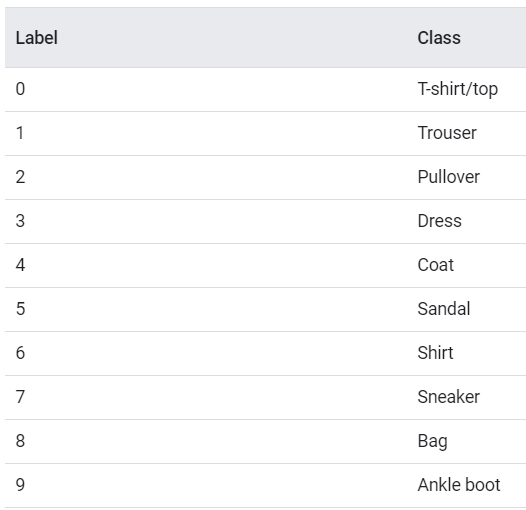``````# 匯入 Fashion MNIST 女人10個用品 訓練資料
``````

## 為什麼第一層完全連接層(Dense)要設為 128?

``````  tf.keras.layers.Dense(32, activation='relu'),

tf.keras.layers.Dense(128, activation='relu'),

tf.keras.layers.Dense(512, activation='relu'),

``````

## 為什麼執行週期(Epoch)要設為 5?

``````# 訓練
history = model.fit(x_train_norm, y_train, epochs=5, validation_split=0.2)

history = model.fit(x_train_norm, y_train, epochs=10, validation_split=0.2)

history = model.fit(x_train_norm, y_train, epochs=20, validation_split=0.2)

``````

## activation function、優化器(optimizer)、損失函數(loss)、效能衡量指標(metrics) 要如何選擇?

1. activation function 有很多種，可參考【維基百科】，可參考中間的表格，包括公式及機率密度函數。早期隱藏層的activation function 大都採取 Sigmoid 函數，但近年發現使用 ReLU，效果比較好。Tensorflow 支援的activation function 可參考【這裡】。範例請參考 04_04_activation_function.ipynb，例如，sigmoid介於 (0,1)，tanh介於 (-1,1)，各有各的應用時機。

2. 優化器(optimizer) 有很多種如下，可參考【這裡】

• SGD
• RMSprop
• Ftrl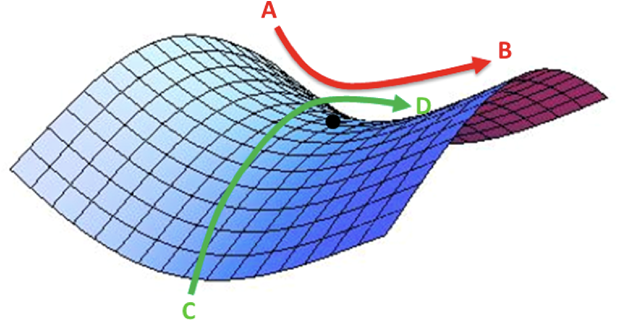1. 損失函數(loss)的介紹，可參考【這裡】
損失函數牽涉到優化求解的收斂的效率，常用的損失函數有均方誤差(Mean Squared Erro, MSE)、交叉熵(Cross entropy)，也可以自訂損失函數，這很重要，很多演算法都是自訂損失函數，產生各種耀眼的成果，例如風格轉換GAN

2. 效能衡量指標(metrics)的介紹，可參考【這裡】。除了準確率(accuracy)外，還包括AUC、Precsion、Recall...等，可在不同的情況下選用，例如，二分類、多分類或不平衡樣本(Imbalanced data)等。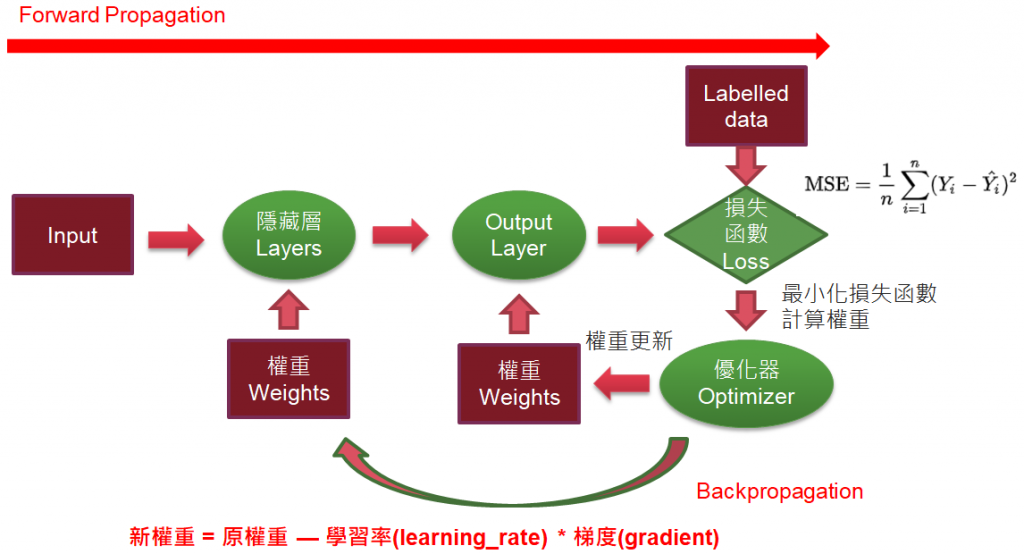## 效能調校(Performance Tuning)的技巧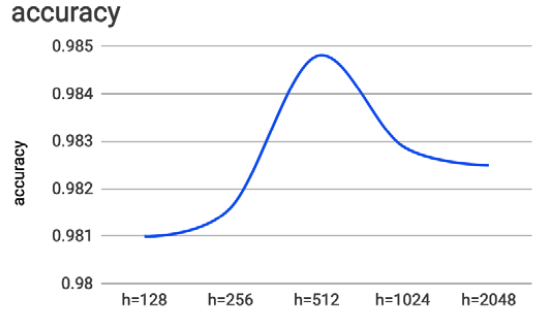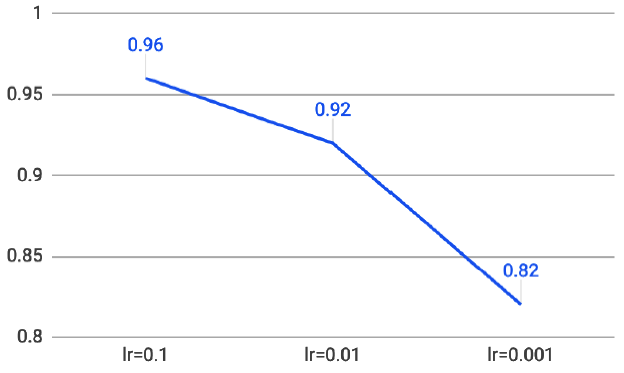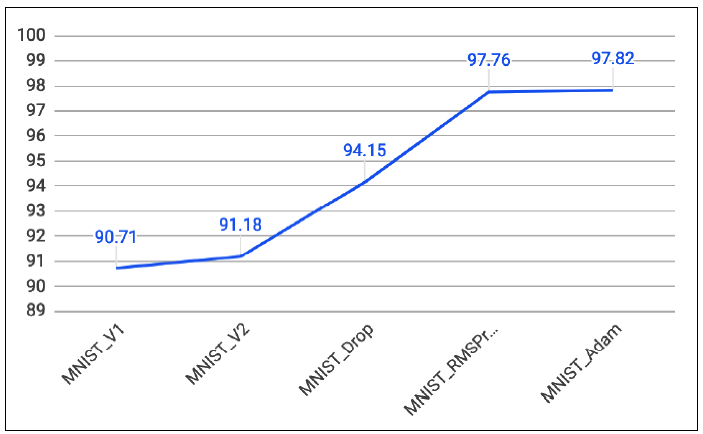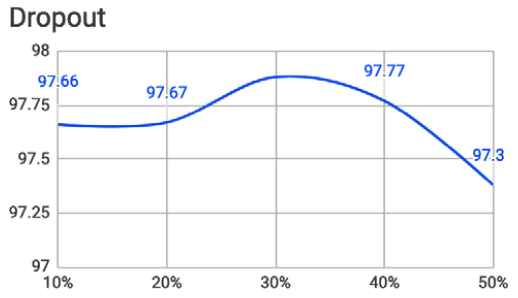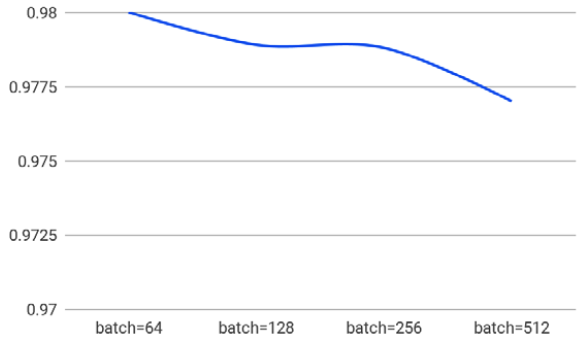``````# 訓練
history = model.fit(x_train_norm, y_train, epochs=5, validation_split=0.2, batch_size=800)
``````

1. 04_01_More_Dense.ipynb
2. 04_02_More_Dense_Fashion_mnist.ipynb
3. 04_03_change_neural_count.ipynb
4. 04_04_change_epoch.ipynb
5. 04_05_activation_function.ipynb